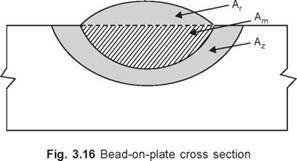## WELDING POWER-SOURCE SELECTION CRITERIA

The following factors must be considered when selecting a power source for welding.

1. Initial cost.

2. Cost of periodic maintenance and repair.

3. Mains supply available: 220 V, 440 V or not available.

4. Steady output current even with input voltage fluctuation.

5. Whether machine causes imbalance in the power load.

6. Machine’s inherent power factor or needs capacitor to raise it.

7. Whether portability is needed.

8. Type of current needed a. c or d. c. or both.

9. Current rating required to accomodate all sizes of electrodes needed for the jobs

10. Machine’s ability to strike and maintain stable arc for the type of electrodes to be

used.

11. Type of volt-ampere characteristics (constant current or constant voltage) needed for the process employed.

12. Whether machine is required to give radiographic quality welds and impact strength with the type of electrodes used.

13. Whether the machine needs to serve several welding processes expected to be used in the shop.

14. Need for remote current control.

15. Machine’s ability to stand shop environment (corrosive gases, moisture, dust, etc.).

3.4 WELDING ENERGY INPUT

3.5.1 Arc Energy Input

The energy input, “H”, is computed as the ratio of total input power, P, of the heat source in watts to its travel velocity, ‘V’, in mm/second.

h = V...(3.1)

If the source of heat is an electric arc

EI

H = - ...(3.2)

where E = voltage in volts and I = current in amperes.

Precisely speaking, net energy input would be

 Hnet =- ...(3.3)

fi_EI

V

where, f1 = the heat transfer efficiency which is from 80% to 90% for most consumable electrode arcs.

The primary function of the heat sources is to melt metal. In this regard it is useful to introduce the concept of melting efficiency, f2, which is the ratio of energy used for melting metal to the total energy supplied.

 f2 =
 ..(3.4)

QAw = QAw. y Hnet fi El

where, Q = theoretical quantity of heat required to melt a given volume of metal. This is

required to elevate the temperature of the solid metal to its melting point plus the heat of

fusion to convert solid to liquid at the melting point.

A reasonable approximation of Q is

Q = (Tm + 273)2/300,000 J/mm3 where, Tm = melting temperature, °C...(3.5)

Aw = Am + Ar...(3.6)

Am = plate cross-section melted Ar = filler metal cross-section melted A, = total weld metal cross-section melted...(3.7)
 A,, =

fi f2 El QV

Let us take the example of submerged arc welding, when an arc weld is made on steel plate under the following conditions:

E = 20 V f1 = 0.9

I = 200 A f2 = 0.3

V = 5 mm/s Q = 10 J/mm3

The weld cross-sectional area-can be estimated on the basis of equation (3.7)

 A,, =

0. 9 x 0.3 x 20 x 200

5 x 10

21.6 mm2

Комментарии закрыты.